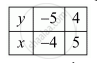Advertisement Remove all ads

# A Two-digit Number is Such that the Product of Its Digits is 20. If 9 is Added to the Number, the Digits Interchange Their Places. Find the Number. - Mathematics

Definition

A two-digit number is such that the product of its digits is 20. If 9 is added to the number, the digits interchange their places. Find the number.

Advertisement Remove all ads

#### Solution

Let the digits at units and tens place of the given number be x and y respectively. Thus, the number is 10y + x.

The product of the two digits of the number is 20. Thus, we have  xy =20

After interchanging the digits, the number becomes 10x + y.

If 9 is added to the number, the digits interchange their places. Thus, we have

(10y+x)+9=10x+y

⇒ 10y +x +9 =10x +y

 ⇒ 1=x + y -10y -x =9

 ⇒ 9x -9y =9

⇒ 9( x - y)=9

 ⇒ x -y 9/9

 ⇒ x - y =1

So, we have the systems of equations

xy=20

x-y=1

Here x and y are unknowns. We have to solve the above systems of equations for xand y.

Substituting  x =1+y from the second equation to the first equation, we get

(1+y )y=20

⇒ y + y^2 = 20

⇒ y^2 + y = 20 =0

 ⇒ y^2 + 5y -4y -20 =0

⇒ y (y + 5)-4(4+5)=0

⇒ ( y + 5)(y - 4)=0

⇒ y = -5 Or y = 4

Substituting the value of in the second equation, we haveHence, the number is 10 xx4 + 5= 45.

Note that in the first pair of solution the values of x and y are both negative. But, the digits of the number can’t be negative. So, we must remove this pair.

Is there an error in this question or solution?
Advertisement Remove all ads

#### APPEARS IN

RD Sharma Class 10 Maths
Chapter 3 Pair of Linear Equations in Two Variables
Exercise 3.7 | Q 12 | Page 86
Advertisement Remove all ads

#### Video TutorialsVIEW ALL 

Advertisement Remove all ads
Share
Notifications

View all notifications

Forgot password?
Course